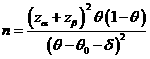# Sample Size Calculator: One-Sample Proportion

Hypothesis: One-Sided Non-Inferiority / SuperiorityData Input: (Help) (Example)

 Input Results α β θ N θ0 δ

Note:

 Variables Descriptions α One-sided significance level 1-β Power of the test θ Expected success proportion of sample θ0 Known success proportion δ True difference of mean response rates, δ>0, the superiority margin or value of δ<0, the non-inferiority margin N Sample size

Help Aids

Application: To test non-inferiority and superiority that unified by the following hypotheses:Here δ is the non-inferiority (δ<0) or superiority margin (δ>0).

Procedure:

1. Enter

a)      value of α, the probability of type I error

b)      value of β, the probability of type II error

c)      value of θ, a true mean response rate of a test drug

d)     value of θ0, a reference response rate

e)      value of δ, the non-inferiority margin (δ<0) or superiority margin (δ>0).

1. Click the button “Calculate” to obtain result sample size N.

Formula:(*)

Notations:

α:               The probability of type I error (significance level) is the probability of rejecting the true null hypothesis.

β:               The probability of type II error (1 – power of the test) is the probability of failing to reject the false null hypothesis.

θ-θ0:          The difference between the true mean response rates of a test drug (θ) and a reference value (θ0).

δ:               Clinically meaningful difference. The largest change from the reference value (baseline) that is considered to be trivial.

Examples

Example 1: We want to show that the majority of patients whose change in bone density after treatment is at least as good as the reference value, say, θ0=30%. If we assume that a difference of 10% in responder rate is considered of no clinical significance, say, δ=-10%, to test for non-inferiority. Also the true response rate is θ=50%. According to (*), at α=0.05, the required sample size for having an 80% power (i.e., β=0.2) is N=18.

Reference:

1. Casagrande, Pike and Smith (1978), Biometrics 34: 483-486.
2. Chow, Shao and Wang, Sample Size Calculations In Clinical Research, Taylor & Francis, NY. (2003) Pages 83-84.
3. Flesis J.L., Statistical Methods for Rates and Proportions (2nd edition). Wiley: New York, 1981.

Top